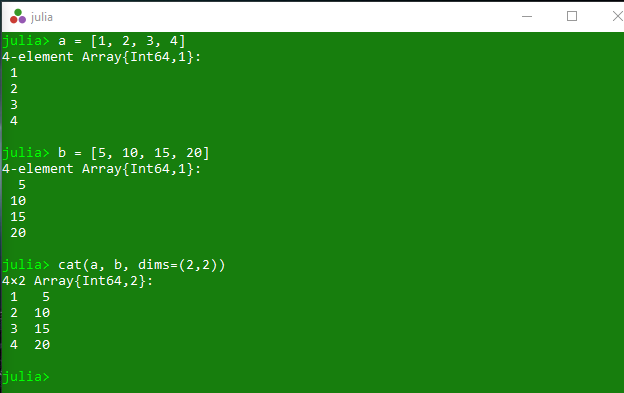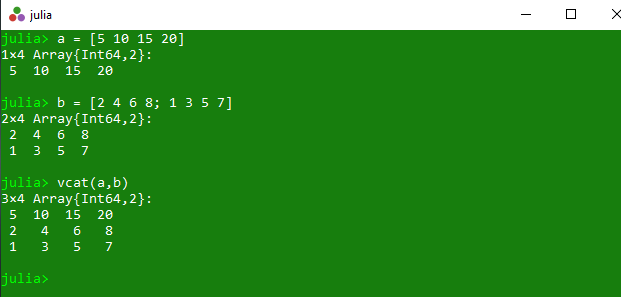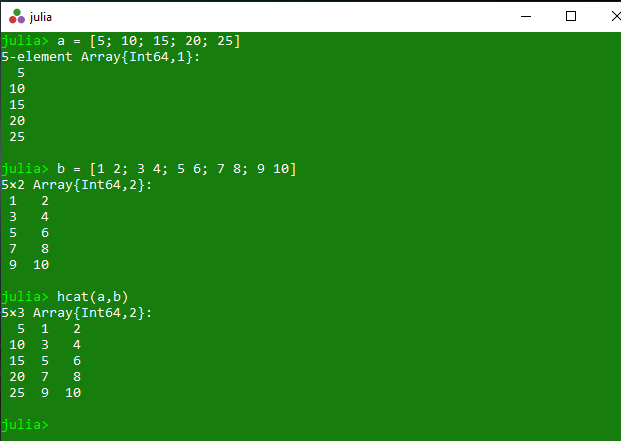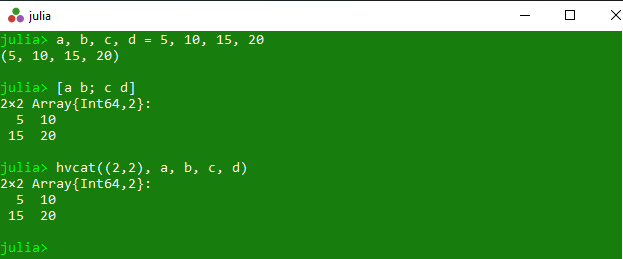Related Articles
Concatenation of arrays in Julia – cat(), vcat(), hcat() and hvcat() Methods
• Last Updated : 01 Apr, 2020

The `cat()` is an inbuilt function in julia which is used to concatenate the given input arrays along the specified dimensions in the iterable dims.

Syntax: cat(A…; dims=dims)

Parameters:

• A: Specified arrays.
• A: Specified dimensions.

Returns: It returns the concatenated array.

Example:

 `# Julia program to illustrate ``# the use of cat() method``  ` `# Getting the concatenated array``a ``=` `[``1``, ``2``, ``3``, ``4``]``b ``=` `[``5``, ``10``, ``15``, ``20``]``cat(a, b, dims ``=``(``2``, ``2``))`

Output:#### vcat()

The `vcat()` is an inbuilt function in julia which is used to concatenate the given arrays along dimension 1.

Syntax: vcat(A…)

Parameters:

• A: Specified arrays.

Returns: It returns the concatenated array.

Example:

 `# Julia program to illustrate ``# the use of vcat() method``  ` `# Getting the concatenated array``a ``=` `[``5` `10` `15` `20``]``b ``=` `[``2` `4` `6` `8``; ``1` `3` `5` `7``]``vcat(a, b)`

Output:#### hcat()

The `hcat()` is an inbuilt function in julia which is used to concatenate the given arrays along dimension 2.

Syntax: hcat(A…)

Parameters:

• A: Specified arrays.

Returns: It returns the concatenated array.

Example:

 `# Julia program to illustrate ``# the use of hcat() method``  ` `# Getting the concatenated array``a ``=` `[``5``; ``10``; ``15``; ``20``; ``25``]``b ``=` `[``1` `2``; ``3` `4``; ``5` `6``; ``7` `8``; ``9` `10``]``hcat(a, b)`

Output:#### hvcat()

The `hvcat()` is an inbuilt function in julia which is used to concatenate the given arrays horizontally and vertically in one call. The first parameter specifies the number of arguments to concatenate in each block row.

Syntax:
hvcat(rows::Tuple{Vararg{Int}}, values…)

Parameters:

• rows: Specified block row.
• values: Specified values.

Returns: It returns the concatenated array.

Example:

 `# Julia program to illustrate ``# the use of hvcat() method``  ` `# Getting the concatenated array``a, b, c, d ``=` `5``, ``10``, ``15``, ``20``[a b; c d]``hvcat((``2``, ``2``), a, b, c, d)`

Output:Attention reader! Don’t stop learning now. Get hold of all the important DSA concepts with the DSA Self Paced Course at a student-friendly price and become industry ready.

My Personal Notes arrow_drop_up